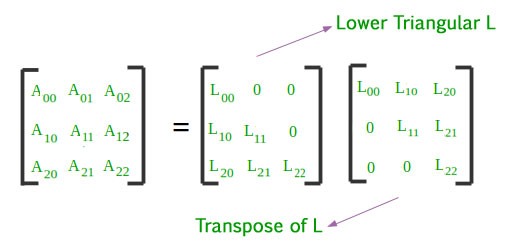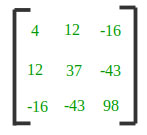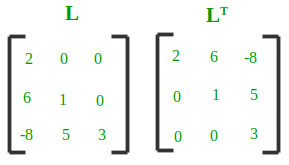# Cholesky Decomposition : Matrix Decomposition

In linear algebra, a matrix decomposition or matrix factorization is a factorization of a matrix into a product of matrices. There are many different matrix decompositions. One of them is Cholesky Decomposition.

The Cholesky decomposition or Cholesky factorization is a decomposition of a Hermitian, positive-definite matrix into the product of a lower triangular matrix and its conjugate transpose. The Cholesky decomposition is roughly twice as efficient as the LU decomposition for solving systems of linear equations.

The Cholesky decomposition of a Hermitian positive-definite matrix A is a decomposition of the form A = [L][L]T, where L is a lower triangular matrix with real and positive diagonal entries, and LT denotes the conjugate transpose of L. Every Hermitian positive-definite matrix (and thus also every real-valued symmetric positive-definite matrix) has a unique Cholesky decomposition.Every symmetric, positive definite matrix A can be decomposed into a product of a unique lower triangular matrix L and its transpose: A = L LT

The following formulas are obtained by solving above lower triangular matrix and its transpose. These are the basis of Cholesky Decomposition Algorithm :Example :

Input :Output :## Recommended: Please try your approach on {IDE} first, before moving on to the solution.

Below is the implementation of Cholesky Decomposition.

## C++

 // CPP program to decompose a matrix using  // Cholesky Decomposition  #include  using namespace std;     const int MAX = 100;     void Cholesky_Decomposition(int matrix[][MAX],                                         int n)  {      int lower[n][n];      memset(lower, 0, sizeof(lower));         // Decomposing a matrix into Lower Triangular      for (int i = 0; i < n; i++) {          for (int j = 0; j <= i; j++) {              int sum = 0;                 if (j == i) // summation for diagnols              {                  for (int k = 0; k < j; k++)                      sum += pow(lower[j][k], 2);                  lower[j][j] = sqrt(matrix[j][j] -                                          sum);              } else {                     // Evaluating L(i, j) using L(j, j)                  for (int k = 0; k < j; k++)                      sum += (lower[i][k] * lower[j][k]);                  lower[i][j] = (matrix[i][j] - sum) /                                        lower[j][j];              }          }      }         // Displaying Lower Triangular and its Transpose      cout << setw(6) << " Lower Triangular"            << setw(30) << "Transpose" << endl;      for (int i = 0; i < n; i++) {                     // Lower Triangular          for (int j = 0; j < n; j++)              cout << setw(6) << lower[i][j] << "\t";          cout << "\t";                     // Transpose of Lower Triangular          for (int j = 0; j < n; j++)              cout << setw(6) << lower[j][i] << "\t";          cout << endl;      }  }     // Driver Code  int main()  {      int n = 3;      int matrix[][MAX] = { { 4, 12, -16 },                          { 12, 37, -43 },                          { -16, -43, 98 } };      Cholesky_Decomposition(matrix, n);      return 0;  }

## Java

 // Java program to decompose   // a matrix using Cholesky  // Decomposition     class GFG  {         //static int MAX = 100;  static void Cholesky_Decomposition(int[][] matrix,                                  int n)  {      int[][] lower = new int[n][n];         // Decomposing a matrix       // into Lower Triangular      for (int i = 0; i < n; i++)      {          for (int j = 0; j <= i; j++)          {              int sum = 0;                 // summation for diagnols              if (j == i)               {                  for (int k = 0; k < j; k++)                      sum += (int)Math.pow(lower[j][k], 2);                  lower[j][j] = (int)Math.sqrt(matrix[j][j] -                                                      sum);              }                              else             {                     // Evaluating L(i, j)                   // using L(j, j)                  for (int k = 0; k < j; k++)                      sum += (lower[i][k] *                               lower[j][k]);                  lower[i][j] = (matrix[i][j] - sum) /                                      lower[j][j];              }          }      }         // Displaying Lower       // Triangular and its Transpose      System.out.println(" Lower Triangular\t Transpose");      for (int i = 0; i < n; i++)       {                     // Lower Triangular          for (int j = 0; j < n; j++)              System.out.print(lower[i][j] + "\t");          System.out.print("");                     // Transpose of           // Lower Triangular          for (int j = 0; j < n; j++)              System.out.print(lower[j][i] + "\t");          System.out.println();      }  }     // Driver Code  public static void main(String[] args)  {      int n = 3;      int[][] matrix = new int[][]{{4, 12, -16},                      {12, 37, -43},                      {-16, -43, 98}};                             Cholesky_Decomposition(matrix, n);  }  }     // This code is contributed by mits

## Python3

 # Python3 program to decompose   # a matrix using Cholesky   # Decomposition  import math  MAX = 100;     def Cholesky_Decomposition(matrix, n):         lower = [[0 for x in range(n + 1)]                   for y in range(n + 1)];         # Decomposing a matrix      # into Lower Triangular      for i in range(n):           for j in range(i + 1):               sum1 = 0;                 # sum1mation for diagnols              if (j == i):                   for k in range(j):                      sum1 += pow(lower[j][k], 2);                  lower[j][j] = int(math.sqrt(matrix[j][j] - sum1));              else:                                     # Evaluating L(i, j)                  # using L(j, j)                  for k in range(j):                      sum1 += (lower[i][k] *lower[j][k]);                  if(lower[j][j] > 0):                      lower[i][j] = int((matrix[i][j] - sum1) /                                                 lower[j][j]);         # Displaying Lower Triangular      # and its Transpose      print("Lower Triangular\t\tTranspose");      for i in range(n):                      # Lower Triangular          for j in range(n):              print(lower[i][j], end = "\t");          print("", end = "\t");                     # Transpose of          # Lower Triangular          for j in range(n):              print(lower[j][i], end = "\t");          print("");     # Driver Code  n = 3;  matrix = [[4, 12, -16],            [12, 37, -43],            [-16, -43, 98]];  Cholesky_Decomposition(matrix, n);     # This code is contributed by mits

## C#

 // C# program to decompose   // a matrix using Cholesky  // Decomposition  using System;     class GFG  {         //static int MAX = 100;  static void Cholesky_Decomposition(int[,] matrix,                                     int n)  {      int[,] lower = new int[n, n];         // Decomposing a matrix       // into Lower Triangular      for (int i = 0; i < n; i++)      {          for (int j = 0; j <= i; j++)          {              int sum = 0;                 // summation for diagnols              if (j == i)               {                  for (int k = 0; k < j; k++)                      sum += (int)Math.Pow(lower[j, k], 2);                  lower[j, j] = (int)Math.Sqrt(matrix[j, j] -                                                        sum);              }                              else             {                     // Evaluating L(i, j)                   // using L(j, j)                  for (int k = 0; k < j; k++)                      sum += (lower[i, k] *                               lower[j, k]);                  lower[i, j] = (matrix[i,j] - sum) /                                         lower[j, j];              }          }      }         // Displaying Lower       // Triangular and its Transpose      Console.WriteLine("  Lower Triangular\t   Transpose");      for (int i = 0; i < n; i++)       {                     // Lower Triangular          for (int j = 0; j < n; j++)              Console.Write(lower[i, j] + "\t");          Console.Write("");                     // Transpose of           // Lower Triangular          for (int j = 0; j < n; j++)              Console.Write(lower[j, i] + "\t");          Console.WriteLine();      }  }     // Driver Code  static int Main()  {      int n = 3;      int[,] matrix = {{4, 12, -16},                       {12, 37, -43},                       {-16, -43, 98}};                              Cholesky_Decomposition(matrix, n);      return 0;  }  }     // This code is contributed by mits

## PHP

 

Output:


Lower Triangular                   Transpose
2         0         0             2         6        -8
6         1         0             0         1         5
-8         5         3             0         0         3


References: Wikipedia – Cholesky decomposition
This article is contributed by Shubham Rana. If you like GeeksforGeeks and would like to contribute, you can also write an article using contribute.geeksforgeeks.org or mail your article to contribute@geeksforgeeks.org. See your article appearing on the GeeksforGeeks main page and help other Geeks.

My Personal Notes arrow_drop_up

Improved By : Mithun Kumar

Article Tags :
Practice Tags :

Be the First to upvote.

Please write to us at contribute@geeksforgeeks.org to report any issue with the above content.